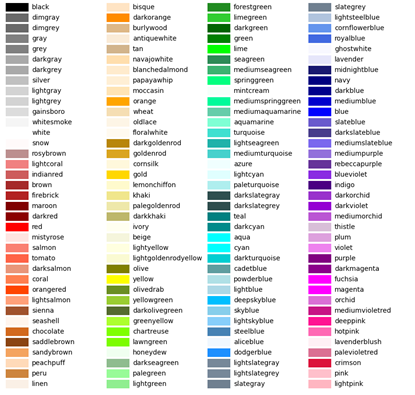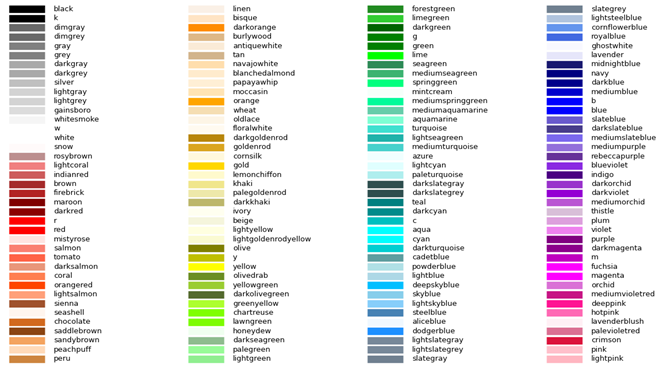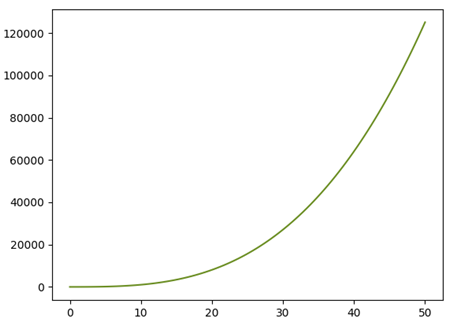# Matplotlib Color Palette

5/5 - (1 vote)

In this article, we’ll learn how to generate the Matplotlib color palette and then we will use it to select a specific color for our plot.

## Problem and Solution Overview

Problem: When presenting data, the color that you assign to a plot is very important; a bad color choice can make your data difficult to understand or even less interesting.

However, it is not easy to choose the right colors or the optimal color combinations for the cases in which we have to plot multiple functions at the same time.

Solution: A solution to this problem is to check the Matplotlib color palette and choose from there, the color that best fits your needs.

We’ll write a script for generating the Matplotlib color palette inside our script, just before plotting our graphs.

In this way, we will be able to check for the name of the color that is most suited for our needs and assign it to a second plot.

We write the code for generating the color palette as a function; to make it possible to call it every time we need to decide on a particular color to assign to the plots.

## Code Overview

We’ll discuss the following code in this tutorial, in a simple to understand, step-by-step manner:

```import matplotlib.pyplot as plt
from matplotlib import colors as mcolors
import numpy as np

def sel_color():

colors = dict(mcolors.BASE_COLORS, **mcolors.CSS4_COLORS)

# sort colors by hue, saturation and value
by_hsv = []
for name, color in colors.items():
t = tuple(mcolors.rgb_to_hsv(mcolors.to_rgba(color)[:3]))
by_hsv.append((t, name))
by_hsv.sort()
sorted_names = [name for hsv, name in by_hsv]

n = len(sorted_names)
ncols = 4
nrows = n // ncols

# Create the matplotlib window
fig, ax = plt.subplots()

# Get height and width of the figure
X, Y = fig.get_dpi() * fig.get_size_inches()

# height of a cell
h = Y / (nrows + 1)

# width of a cell
w = X / ncols

# using an index for accessing all the color names
k = 0

# inserting the colors in the palette by column
for i in range(ncols):
for j in range(nrows, 0, -1):

# current row
row = j * h

# current column
col = i

# height level for the colors' labels
y = row

# position of the initial corner of the color box
xi = w * (col + 0.05)

# position of the final corner of the color box
xf = w * (col + 0.25)

# initial position of the labels
xi_text = w * (col + 0.3)

ax.text(xi_text, y, sorted_names[k], fontsize=(h * 0.8),
horizontalalignment='left', verticalalignment='center')

ax.hlines(y, xi, xf, color=sorted_names[k], linewidth=(h * 0.8))
k += 1

ax.set_xlim(0, X)
ax.set_ylim(0, Y)
ax.set_axis_off()

top=1, bottom=0,
hspace=0, wspace=0)
plt.show()
selected_c = str(input('Enter the name of the color for your graph: '))
return selected_c

# x and y array to plot
x = np.linspace(0, 50, 100)
y = x**3 + 3*x - 5

# Plotting
current_c = sel_color()
fig = plt.figure()
ax = fig.subplots()
ax.plot(x, y, color=current_c)
plt.show()
```

If you run this code snippet, you’ll get the following output:

You can resize it accordingly to get a more pretty output using Python’s internal display window tool.

Let’s dive into the code!

## Importing Libraries and Packages

For this example, we just need `Matpotlib `and `Numpy`.

In addition to `.pyplot()`, which is used for plotting, we also need the module `colors` that contains functions and classes for color specification and conversion. We then call it “`mcolors`”.

🌍 Link: You can find the full documentation here

Numpy is used just for generating two random arrays that will be then plotted, after choosing the color that we choose from the generated palette.

```import matplotlib.pyplot as plt
from matplotlib import colors as mcolors
import numpy as np
```

## Generating the Matplotlib Color Palette

As anticipated in the introduction, the aim of this section is to generate the Matplotlib color palette and display it in a Matplotlib window.

For those who are not familiar with the color palettes that can be used in Python, the following figure shows the Matplotlib color palette that we want to recreate in our script:Figure 1: Color palette containing all the names of the colors that can be used within a matplotlib window.

## Defining the Function and Sorting the Colors by HSV Values

As anticipated in the introduction, we write this script as a portable function to generate the color palette. Portability means that you can reuse the same function in other scripts just by calling the function name!

We start by calling the function `sel_col()`.

Within the body of the function, we create a dictionary that contains all the names of the colors (the basic ones and the CSS colors) and the tuple or the hexadecimal codes that univocally describe a particular color, as `key:value` pairs; we assign the dictionary to the variable `colors`.

```def sel_color():
colors = dict(mcolors.BASE_COLORS, **mcolors.CSS4_COLORS)
```

As you might see by printing the variable `colors`, the basic colors are defined by a tuple of three values while the other colors are represented by a hexadecimal code.

In order to adopt a uniform nomenclature for the description of each color, we will convert this representation first to an RGBA.

💡 Info: RGBA is a possible way to describe a color with 4 numbers, the first three correspond to the amount of Red, Green, and Blue. The fourth one stands for intensity.

Next, we convert it to an hexadecimal.

The first step can be done by exploiting the function `mcolors.to_rgba()`, which takes as input the array containing all the tuples representing the different colors.

The second step instead, can be done with the function `mcolors.rgb_to_hsv()` that takes as input the tuples corresponding to the RGB description of the colors and converts it into HSV format, which stands for Hue, Saturation, and Value (or Brightness).

We select just the first three values since we do not want to apply any change to the intensity, which moreover, is not provided in the RGB color code.

We store each HSV combination of numbers into a tuple, together with the respective color name and then extend this procedure to all the colors listed in `colors` and hence generate a list of these tuples.

Since `colors` is a dictionary, to access both its keys and values we write: `for name, color in colors.items()` in this way the variables `name` and `color` refer to the dictionary keys and values, respectively.

To conclude, we sort the list by using the function `list.sort()`, passing as input the whole list and we store all of the HSV color tuples into the variable `by_hsv`.

The following code lines display all the aforementioned procedures.

```    # sort colors by hue, saturation and value
by_hsv = []
for name, color in colors.items():
t = tuple(mcolors.rgb_to_hsv(mcolors.to_rgba(color)[:3]))
by_hsv.append((t, name))
by_hsv.sort()```

If we now print the variable `by_hsv`, we can see that all the colors have been sorted by their HSV values, from the lowest to the highest.

We finish this section by creating a list containing all the names of the colors. To do that, we exploit list comprehension and we store in the list `sorted_names` just the name of the colors present in the tuples of the `by_hsv` list.

`  sorted_names = [name for hsv, name in by_hsv]`

## Creating the Color Palette Framework

At this point, we create the palette that will be displayed in the Matplotlib window.

We start by defining the number of rows and columns. As you can see from the previous figure, we want to have four columns; the number of rows is defined by the ratio between the total number of colors (equal to the length of the list `sorted_names`) and the number of columns.

```  n = len(sorted_names)
ncols = 4
nrows = n // ncols
```

At this point we create the Matplotlib window, exploiting the Matplotlib function `subplots()`.

```  # Create the matplotlib window
fig, ax = plt.subplots()```

As you can see from Figure 1, the colors appear in a grid-like framework that uniformly occupies the window.

In order to do that, we have to extract the size of our window and thus define the size that each colored box and its respective label should occupy.

To obtain the height and the width of the figure, we combine together the methods `.get_dpi()` returning the resolution in dots per inch as a float number and `.get_size_inches()` returning the size of the figure in inches.

🌍 Resources: Documentation of those two methods can be found here and here.

By multiplying them together, we hence obtain the size of the figure in dots.

We assign the width and the height values to the variables `X` and `Y`.

After that, we calculate the height and width of each row and column (`h` and `w`, respectively) by dividing `Y` and `X` by the total number of rows and columns (note that for `h`, we divide `Y` by `nrows +1` to obtain a little more space on the upper and lower corners of the window).

```  X, Y = fig.get_dpi() * fig.get_size_inches()
# height of a cell
h = Y / (nrows + 1)
# width of a cell
w = X / ncols
```

## Inserting the Colored Boxes in the Palette

### Position and size of the colored boxes and labels

Now that we calculated the height and width of the `cells` of our palette, we can proceed further by inserting in each position the colored boxes and their respective names.

Before accessing the cells in which the figure is “divided” — actually, the figure is not divided into cells, it is just a way of thinking about that, each cell corresponds to a colored box plus its label — we define a parameter `k` for indexing the names of the colors in the `sorted_names` list.

```  # using an index for accessing all the color names
k = 0
```

At this point, we can access each position of the figure and create all the colored boxes.

In order to do that, we exploit two nested `f`or loops that will allow us to insert each color in its position.

We start by first filling the first column, then we proceed with the second one, and so on till the fourth one.

The first `for` loop refers to the columns and will iterate throughout all of them. We specify this by setting the end value `ncols` equal to four.

On the other hand, the nested `for` loop is responsible for the rows. Since we want to start creating our boxes from the upper corner, this `for` loop has to iterate in the reversed order, so the first index will correspond to the last row (specified by the value `nrows`) and the final index will be 0, which identifies the row at the bottom of the figure.

Within this second `for` loop, we firstly define two parameters: `row` and `col`, which identify the row and the column we are currently working on, respectively.

As you can see, `col` is equal to the index of the outer for loop while `row` is defined by multiplying the height of a single cell, `h`, by the index of the inner `for` loop (which is iterating through all the rows).

The parameter `row` will be also responsible for the height level of the colors’ labels.

```  # inserting the colors in the palette by column
for i in range(ncols):
for j in range(nrows, 0, -1):
# current row
row = j * h
# current column
col = i
# height level for the colors' labels
y = row
```

Now that we can access each position of the figure in the correct order, we start to create the colored boxes.

The first thing to do is to specify the size of the boxes, we do that by defining the initial and final horizontal coordinate of the boxes, we call them “xi” and “xf”, respectively.

As you can see in the code below, “xi” is defined by the cell width, multiplied by the current column position (“col”) to which we also add a small offset (0.05); for “xf”, the procedure is similar, its position has just a different offset, 0.25 instead of 0.05.

From this, you can clearly understand that the width of our boxes will be 0.20. The starting position of the labels, “xi_text” is defined in the same way, just with another offset, this time 0.3.

```         # position of the initial line of the colored square
xi = w * (col + 0.05)
# position of the final line of the colored square
xf = w * (col + 0.25)
# initial position of the text
xi_text = w * (col + 0.3)
```

Once we defined the initial and final position of the edges of the boxes and of the text labels, we can finally create them.

To generate the labels, we exploit the function ax.text(), which generates some axes in which we can insert a text.

We have to specify, as input parameter, the horizontal and vertical position (“xi_text” and “y”, respectively), the label (`sorted_names[k]`).

We can also specify some other less important and optional parameters, like the font size and the position of the labels with respect to the initial coordinates.

## Properties of the Colored Boxes and Their Labels

```       ax.text(xi_text, y, sorted_names[k], fontsize=(h * 0.8),
horizontalalignment='left', verticalalignment='center')```

To create the boxes, we exploit the function ax.hlines(), which generates an horizontal line.

This time we first specify the vertical coordinate “y”, then the starting and ending point of the line, “xi” and “xf”, respectively; we also specify the color of the line by accessing the name of the colors (using “sorted_names[k]”) and the line width, which corresponds to the height of the box ( ̴ 80% of the cell height).

We end this for loop by incrementing the value of the index “k”; in this way, in the next iteration, we will change `row` and hence we will be addressing the next color name in the list “`sorted_names`”.

```       ax.hlines(y, xi, xf,
color=sorted_names[k], linewidth=(h * 0.8))
k += 1```

We are almost done with our function sel_color(), we just conclude by specifying some details regarding the size of the axes in the Matplotlib window and by disabling their visibility.

We also adjust the position of the figure within the Matplotlib window.

In the end, we show the created palette and ask (by using a print statement) to select the name of a color in the palette. The function will return the typed color name, stored in the variable “selected_c”.

```  ax.set_xlim(0, X)
ax.set_ylim(0, Y)
ax.set_axis_off()

top=1, bottom=0,
hspace=0, wspace=0)
plt.show()
selected_c = str(input('Enter the name of the color for your graph: '))
return selected_c```

The palette will be shown as represented in Figure 2, which is very similar to the one we had in Figure 1.Figure 2: Color palette created within the function sel_color() and displaying all the colors available within Matplotlib. As you can see, some colors are repeated but it is just because they can be addressed by typing different names.

## Plotting a Graph with the Selected Color

After having completed the sel_color() function, we create two arrays “x” and “y” and we plot them in another Matplotlib window.

However, before doing that, we call the function sel_color() and assign its returning value (the name of the color that we selected and typed in the terminal, I typed “olivedrab” as an example) to the variable “current_c” which will be inserted as the value of the parameter color, within the ax.plot() function.

The ending result is shown in Figure 3, i.e., a plot that has the color that we chose after checking the just created color palette.Figure 3: “x” and “y” arrays plotted with the color that we selected from the palette of Figure 2.

## Conclusions

In this article, we defined a function for creating and displaying the Matplotlib color palette.

We first defined the size of the palette, its rows and columns.

Then we calculated the size of each cell and by accessing their position (exploiting two nested for loops) we created the colored boxes and their labels.

Finally, we entered in the terminal the name of the color that we selected and called the function in order to assign that specific color to the plot that we wanted to plot.